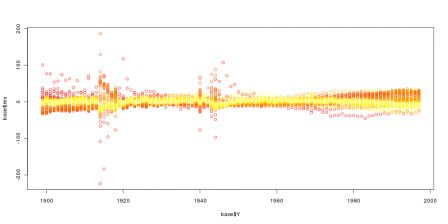Want to share your content on R-bloggers? click here if you have a blog, or here if you don't.

Last course will be uploaded soon (the links will be here and there). The R code considered is given below. First, we had to work a little bit on the datasets,

`tabB=read.table("http://perso.univ-rennes1.fr/arthur.charpentier/tabB.csv", sep=";",header=FALSE) ANNEE=tabB[,1] BASEB=tabB[,seq(2,246,by=2)] BASEB=as.matrix(BASEB[,1:100])AGE=0:ncol(BASEB)tabC=read.table("http://perso.univ-rennes1.fr/arthur.charpentier/tabC.csv", sep=";",header=FALSE) an=tabC[,1]an=an[-c(16,23,43,48,51,53,106)]BASEC=tabC[,2:101] BASEC=as.matrix(BASEC[-c(16,23,43,48,51,53,106),])  BASEB=BASEB[,1:90]BASEC=BASEC[,1:90]AGE=AGE[1:90]MU=as.matrix(log(BASEB/BASEC))persp(ANNEE,AGE,MU,theta=70,shade=TRUE,col="green")library(rgl)persp3d(ANNEE,AGE,MU,col="light blue")`

(this last line is here to play a little bit with the 3d mortality surface). We first used the Lee-Carter function proposed by JPMorgan in LifeMetrics,

`source("http://perso.univ-rennes1.fr/arthur.charpentier/fitModels.r")x=AGEy=ANNEEd=BASEBe=BASECw=matrix(1,nrow(d),ncol(e))LC1=fit701(x,y,e,d,w)plot(AGE,LC1\$beta1)plot(ANNEE,LC1\$kappa2)plot(AGE,LC1\$beta2)`

Then we considered nonlinear Poisson regression,

`D=as.vector(BASEB)E=as.vector(BASEC)A=rep(AGE,each=length(ANNEE))Y=rep(ANNEE,length(AGE))base=data.frame(D,E,A,Y,a=as.factor(A),y=as.factor(Y)) LC2=gnm(D~a+Mult(a,y),offset=log(E),family=poisson,data=base)plot(AGE[-1],LC2\$coefficients+LC2\$coefficients[2:90])lines(AGE,LC1\$beta1,col="blue")plot(ANNEE,LC2\$coefficients[181:279])plot(ANNEE,LC1\$kappa2,col="red")plot(AGE,LC1\$beta2)`

As mentioned during the course, this technique is great… but it is sentive to initial values in the optimization procedure. For instance, consider the following loops,

`plot(AGE[-1],LC2\$coefficients+LC2\$coefficients[2:90],type="l",col="blue",xlab="",ylab="")for(s in 1:200){LC2=gnm(D~a+Mult(a,y),offset=log(E),family=poisson,data=base)lines(AGE[-1],LC2\$coefficients+LC2\$coefficients[2:90],col="blue")}`

Here are representation of the first component in the Lee-Carter model,Hence, the estimation depends on the choice of initial values… even if the shape remains unchanged…
Then, we finnished with Rob Hyndman’s package,

`library(demography)base=demogdata(data=t(exp(MU)),pop=t(BASEC),ages=AGE,years=ANNEE,type="mortality",label="France",name="Total",lambda=0)LC3=lca(base)LC3F=forecast(LC3,100)plot(LC3\$year,LC3\$kt,xlim=c(1900,2100),ylim=c(-300,150))lines(LC3F\$year,LC3\$kt+LC3F\$kt.f\$mean)lines(LC3F\$year,LC3\$kt+LC3F\$kt.f\$lower,lty=2)lines(LC3F\$year,LC3\$kt+LC3F\$kt.f\$upper,lty=2)`

We concluded with a short discussion about errors of the Lee-Carter model (on French mortality)

`D=as.vector(BASEB)E=as.vector(BASEC)A=rep(AGE,each=length(ANNEE))Y=rep(ANNEE,length(AGE))base=data.frame(D,E,A,Y,a=as.factor(A),y=as.factor(Y))RES=residuals(LC2,"pearson")base\$res=RESplot(base\$A,base\$res)couleur=heat.colors(100)plot(base\$A,base\$res,col=couleur[base\$Y-1898])plot(base\$Y,base\$res,col=couleur[base\$A+1])`

The graphs can be seen below (as a function of time, and a function of ages)his Wednesday, we will discuss how we can use those estimators (or other ones) in life insurance…